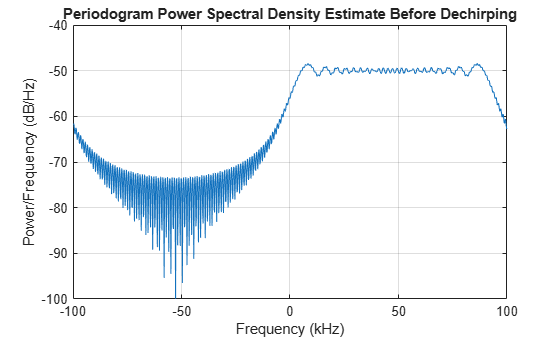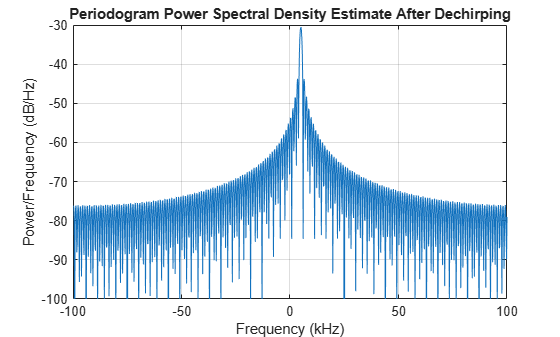# dechirp

Perform dechirp operation on FMCW signal

## Syntax

``y = dechirp(x,xref)``

## Description

example

````y = dechirp(x,xref)` mixes the incoming signal, `x`, with the reference signal, `xref`. The signals can be complex baseband signals. In an FMCW radar system, `x` is the received signal and `xref` is the transmitted signal.```

## Examples

collapse all

Dechirp a delayed FMCW signal, and plot the spectrum before and after dechirping.

Create an FMCW signal.

```Fs = 2e5; Tm = 0.001; hwav = phased.FMCWWaveform('SampleRate',Fs,'SweepTime',Tm); xref = step(hwav);```

Dechirp a delayed copy of the signal.

```x = [zeros(10,1); xref(1:end-10)]; y = dechirp(x,xref);```

Plot the spectrum before dechirping.

```[Pxx,F] = periodogram(x,[],1024,Fs,'centered'); plot(F/1000,10*log10(Pxx)); grid; xlabel('Frequency (kHz)'); ylabel('Power/Frequency (dB/Hz)'); title('Periodogram Power Spectral Density Estimate Before Dechirping');```Plot the spectrum after dechirping.

```[Pyy,F] = periodogram(y,[],1024,Fs,'centered'); plot(F/1000,10*log10(Pyy)); xlabel('Frequency (kHz)'); ylabel('Power/Frequency (dB/Hz)'); ylim([-100 -30]); grid title('Periodogram Power Spectral Density Estimate After Dechirping');```## Input Arguments

collapse all

Incoming signal, specified as an M-by-N matrix. Each column of `x` is an independent signal and is individually mixed with `xref`.

Data Types: `single` | `double`
Complex Number Support: Yes

Reference signal, specified as an M-by-1 vector.

Data Types: `single` | `double`
Complex Number Support: Yes

## Output Arguments

collapse all

Dechirped signal, returned as an M-by-N matrix. Each column is the mixer output for the corresponding column of `x`.

Data Types: `single` | `double`

## Algorithms

For column vectors `x` and `xref`, the mix operation is defined as `xref .* conj(x)`.

If `x` has multiple columns, the mix operation applies the preceding expression to each column of `x` independently.

The mix operation reverses the Doppler shift embedded in `x`, because of the mixing order of `xref` and `x`. The mixing order affects the sign of the imaginary part of the output argument, `y`. There is no consistent convention in the literature about the mixing order. This function and the `beat2range` function use the same convention. If your program processes the output of `dechirp` in other ways, take the mixing order into account.

This function supports single and double precision for input data and arguments. If the input data, `x`, is single precision, the output data is single precision, regardless of the precision of the arguments. If the input data is double precision, the output data is double precision, regardless of the precision of the arguments.

 Pace, Phillip. Detecting and Classifying Low Probability of Intercept Radar. Boston: Artech House, 2009.

 Skolnik, M.I. Introduction to Radar Systems. New York: McGraw-Hill, 1980.